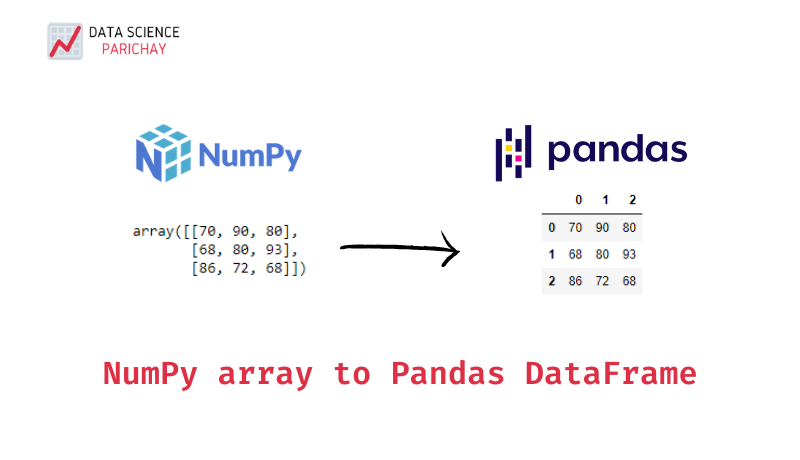# Create Pandas DataFrame from a Numpy Array

Pandas dataframes are quite versatile when it comes to manipulating 2D tabular data in python. And often it can be quite useful to convert a numpy array to a pandas dataframe for manipulating or transforming data. In this tutorial, we’ll look at how to create a pandas dataframe from a numpy array.

To create a pandas dataframe from a numpy array, pass the numpy array as an argument to the `pandas.DataFrame()` function. You can also pass the index and column labels for the dataframe. The following is the syntax:

`df = pandas.DataFrame(data=arr, index=None, columns=None)`

Let’s look at a few examples to better understand the usage of the `pandas.DataFrame()` function for creating dataframes from numpy arrays.

Let’s create a dataframe by passing a numpy array to the `pandas.DataFrame()` function and keeping other parameters as default.

```import numpy as np
import pandas as pd

# sample numpy array
arr = np.array([[70, 90, 80], [68, 80, 93]])
# convert to pandas dataframe with default parameters
df = pd.DataFrame(arr)

# print
print("Numpy array:\n", arr)
print("\nPandas dataframe:\n", df)```

Output:

```Numpy array:
[[70 90 80]
[68 80 93]]

Pandas dataframe:
0   1   2
0  70  90  80
1  68  80  93```

In the above example, the dataframe `df` is created from the numpy array `arr`. Note that since we did not pass the index and column labels, the created dataframe used the default RangeIndex for them.

Let’s pass custom index and column labels to the dataframe being created.

📚 Data Science Programs By Skill Level

Introductory

Intermediate ⭐⭐⭐

🔎 Find Data Science Programs 👨‍💻 111,889 already enrolled

Disclaimer: Data Science Parichay is reader supported. When you purchase a course through a link on this site, we may earn a small commission at no additional cost to you. Earned commissions help support this website and its team of writers.

```import numpy as np
import pandas as pd

# sample numpy array
arr = np.array([[70, 90, 80], [68, 80, 93]])
# convert to pandas dataframe with custom index and column names
df = pd.DataFrame(arr, columns=['History', 'Physics', 'Math'], index=['Sam', 'Emma'])

# print
print("Numpy array:\n", arr)
print("\nPandas dataframe:\n", df)```

Output:

```Numpy array:
[[70 90 80]
[68 80 93]]

Pandas dataframe:
History  Physics  Math
Sam        70       90    80
Emma       68       80    93```

Here, the index labels and column names are passed to the arguments `index` and `columns` respectively. From the labels, we can assume that the dataframe stores the test scores of students `Sam` and `Emma` in the subjects `History`, `Physics` and `Math`.

Passing a one-dimensional numpy array to the `pandas.DataFrame()` function will result in a pandas dataframe with one column.

```import numpy as np
import pandas as pd

# sample numpy array
arr = np.array([10, 20, 30, 40])
# convert to pandas dataframe
df = pd.DataFrame(arr)

# print
print("Numpy array:\n", arr)
print("\nPandas dataframe:\n", df)```

Output:

```Numpy array:
[10 20 30 40]

Pandas dataframe:
0
0  10
1  20
2  30
3  40
```

Fore more on the `pandas.DataFrame()` function, refer to its official documentation.

Pandas dataframes are objects used to store two-dimensional tabular data. If you try to create a pandas dataframe from a numpy array with more than 2 dimensions, you’ll get an error. See the example below.

```import numpy as np
import pandas as pd

# sample numpy array
arr = np.random.randint(1,5,(3,3,2))
print("Numpy array:\n", arr)
# convert to pandas dataframe
df = pd.DataFrame(arr)
print("\nPandas dataframe:\n", df)```

Output:

```Numpy array:
[[[4 4]
[2 2]
[2 2]]

[[2 2]
[4 4]
[1 3]]

[[3 4]
[4 1]
[2 2]]]
---------------------------------------------------------------------------
ValueError                                Traceback (most recent call last)
<ipython-input-12-8c5dce07516e> in <module>
6 print("Numpy array:\n", arr)
7 # convert to pandas dataframe
----> 8 df = pd.DataFrame(arr)
9 print("\nPandas dataframe:\n", df)

~\anaconda3\lib\site-packages\pandas\core\internals\construction.py in prep_ndarray(values, copy)
293         values = values.reshape((values.shape, 1))
294     elif values.ndim != 2:
--> 295         raise ValueError("Must pass 2-d input")
296
297     return values

ValueError: Must pass 2-d input```

* Some lines in the above error message have been skipped to shorten the output shown.

With this, we come to the end of this tutorial. The code examples and results presented in this tutorial have been implemented in a Jupyter Notebook with a python (version 3.8.3) kernel having numpy version 1.18.5 and pandas version 1.0.5

•